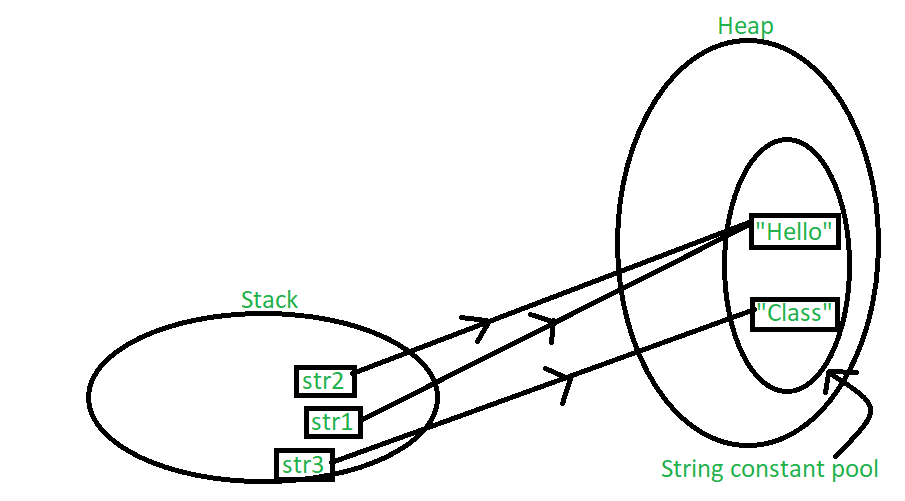Techno Blender
Digitally Yours.
0 21

In this article, we will try to understand how the Strings are stored in JavaScript. Strings are a primitive data type in JavaScript and are allocated a special place in the memory to store and manipulate. The special place allocated in the memory is known as String Constant Pool. The string constant pool is a small cache that resides within the heap. JavaScript stores all the values inside the string constant pool on direct allocation.

In other words, the string constant pool exists mainly to reduce memory usage and improve the reuse of existing instances in memory. There are some cases where we want a distinct String object to be created even though it has the same value. In this case, we use the new keyword.

Let us now understand with a basic example, how strings are stored in memory.

Example 1: In this example, we will create Strings and understand how they are stored in memory.

## Javascript

 `var` `str1 = ``"Hello"``;` `var` `str2 = ``"Hello"``;` `var` `str3 = ``"World"``;` `console.log(str1 == str2);` `console.log(str1 == str3);`

Output:

```true
false```

Below is the diagrammatic representation of how the strings in the above example will be stored in memory.

How are strings stored in javascript?

We can see that str1 and str2 point at the same location in the memory while a new space is created for str3 as it has a different value. In this way string constant pool saves memory by making the same value string point to the same location in the memory.

Example 2: In this example, we will make the strings with the same value refer to different locations in memory

## Javascript

 `var` `str1 = ``new` `String(``"John"``);` `var` `str2 = ``new` `String(``"John"``);` `var` `str3 = ``new` `String(``"Doe"``);` `console.log(str1 == str2);` `console.log(str1 == str3);`

Output:

```false
false```

How are strings stored in javascript?

We can see in the image that even though str1 and str2 are having the same value but because of the new keyword they are referring to different locations in the memory. Hence, they return false when compared.

Conclusion:

When creating the strings using quotations(” “) they are directly stored in the String Constant Pool where equal values refer to the same location in the memory. Whereas, when strings are created using the new keyword, a new instance is always created in the heap memory then the value is stored in the String Constant Pool because of this even if the data stored is the same still the strings will not be equal.

In this article, we will try to understand how the Strings are stored in JavaScript. Strings are a primitive data type in JavaScript and are allocated a special place in the memory to store and manipulate. The special place allocated in the memory is known as String Constant Pool. The string constant pool is a small cache that resides within the heap. JavaScript stores all the values inside the string constant pool on direct allocation.

In other words, the string constant pool exists mainly to reduce memory usage and improve the reuse of existing instances in memory. There are some cases where we want a distinct String object to be created even though it has the same value. In this case, we use the new keyword.

Let us now understand with a basic example, how strings are stored in memory.

Example 1: In this example, we will create Strings and understand how they are stored in memory.

## Javascript

 `var` `str1 = ``"Hello"``;` `var` `str2 = ``"Hello"``;` `var` `str3 = ``"World"``;` `console.log(str1 == str2);` `console.log(str1 == str3);`

Output:

```true
false```

Below is the diagrammatic representation of how the strings in the above example will be stored in memory.How are strings stored in javascript?

We can see that str1 and str2 point at the same location in the memory while a new space is created for str3 as it has a different value. In this way string constant pool saves memory by making the same value string point to the same location in the memory.

Example 2: In this example, we will make the strings with the same value refer to different locations in memory

## Javascript

 `var` `str1 = ``new` `String(``"John"``);` `var` `str2 = ``new` `String(``"John"``);` `var` `str3 = ``new` `String(``"Doe"``);` `console.log(str1 == str2);` `console.log(str1 == str3);`

Output:

```false
false```

How are strings stored in javascript?

We can see in the image that even though str1 and str2 are having the same value but because of the new keyword they are referring to different locations in the memory. Hence, they return false when compared.

Conclusion:

When creating the strings using quotations(” “) they are directly stored in the String Constant Pool where equal values refer to the same location in the memory. Whereas, when strings are created using the new keyword, a new instance is always created in the heap memory then the value is stored in the String Constant Pool because of this even if the data stored is the same still the strings will not be equal.